Demo problem: Flow in a 2D channel with an oscillating wall

The moving-boundary Navier-Stokes problem discussed in this document is a "warm-up" problem for the classical fluid-structure interaction problem of flow in a 2D collapsible channel. Here we compute the flow through a 2D channel in which part of one wall is replaced by a moving "membrane" whose motion is prescribed. In another example, we will demonstrate how easy it is to extend the driver code for the current problem to a fluid-structure interaction problem in which the "membrane" is represented by an elastic beam that deforms in response to the fluid traction.

We note that the (FSI version of the) problem considered here was analysed in much more detail in

• Jensen, O.E. & Heil, M. (2003) High-frequency self-excited oscillations in a collapsible-channel flow. Journal of Fluid Mechanics 481 235-268. (pdf preprint) (abstract)

where a detailed discussion (and an asymptotic analysis) of the flow-structures described below may be found.

# The problem

 Finite-Reynolds-number flow in a 2D channel with an oscillating wall . The figure below shows a sketch of the problem: Flow is driven by a prescribed pressure drop through a 2D channel of width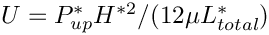and total length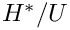The upstream and downstream lengths of the channel are rigid, whereas the upper wall in the central section performs a prescribed oscillation. The shape of the moving segment is parametrised by a Lagrangian coordinate,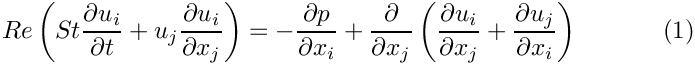, so that the position vector to the moving wall is given by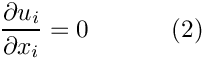.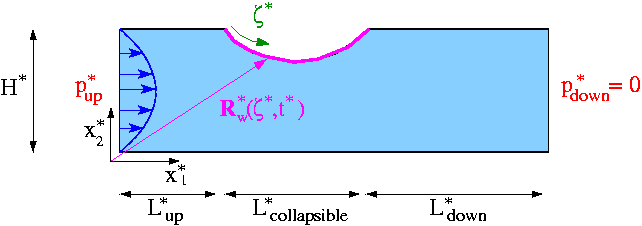Sketch of the problem. We scale all lengths on the channel width,, use the average velocity through the undeformed channel,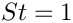, to scale the velocities, and use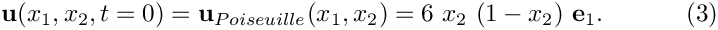to non-dimensionalise time. (As usual, we employ asterisks distinguish dimensional parameters from their non-dimensional equivalents.) The flow is then governed by the unsteady Navier-Stokes equations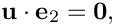and the continuity equation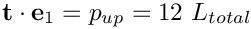with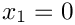, subject to the following boundary and initial conditions: Initial condition: Poiseuille flow, i.e.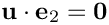Parallel inflow,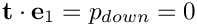and an applied axial traction of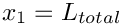at the upstream end,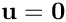. Parallel, axially traction-free outflow at the downstream end, i.e.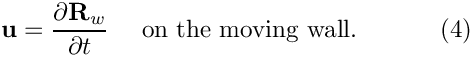and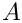at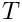. No slip on all channel walls, i.e.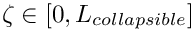on the rigid walls and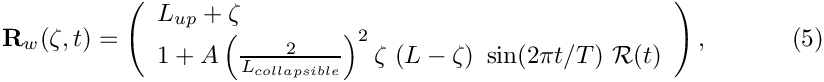We consider a wall motion that deforms the initially "flush" wall into a parabolic shape. We denote the non-dimensional
amplitude of the oscillation by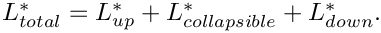and its period by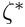, and parametrise the position vector to a point on the wall by the Lagrangian coordinate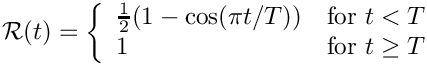as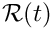where the "ramp" function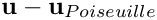is used to facilitate the start-up of the simulation from the initial condition of steady Poiseuille flow.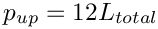provides a "smooth" startup of the wall motion during the first period of the oscillation.

# The results

The figure below shows a snapshot, taken from the animation of the computational results. The first four figures show (from top left to bottom right) "carpet plots" of the axial and transverse velocities, the axial component of the perturbation velocity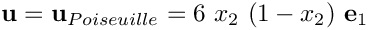, and the pressure distribution. The 2D contour plot at the bottom of the figure shows a contour plot of the pressure and a few instantaneous streamlines.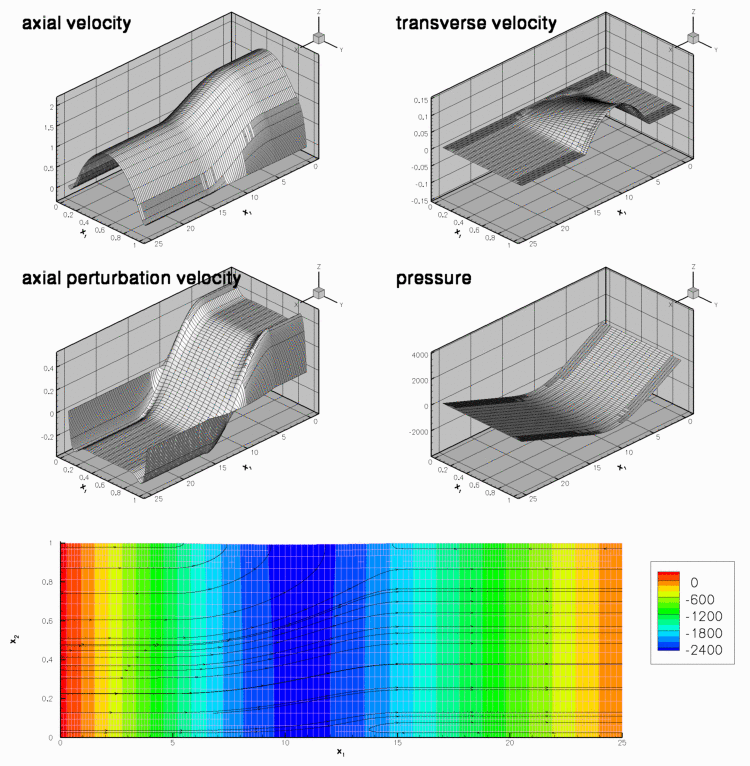Snapshot from the animation of the flow field for Re = Re St = 50, T=0.45, A=0.01.

The figures illustrate the flow structures identified in Jensen & Heil's (2003) asymptotic analysis of 2D channel flows that are driven by high-frequency, small-amplitude oscillations of a finite section of one of their walls: The flow consists of oscillatory axial "sloshing flows", superimposed on the mean Poiseuille flow that is driven by the applied pressure drop. During phases when the wall moves inwards (outwards) the flow generated by the moving wall decelerates (accelerates) the flow in the upstream region as the wall "injects" fluid into ("sucks" fluid out of) the domain. Conversely, in the downstream region the flow generated by the wall adds to the pressure-driven mean flow during phases when the wall moves inwards. This is shown most clearly in the plot of the axial velocity perturbation. In the plots shown above, the wall moves outwards and the axial perturbation velocity is positive (i.e. in the direction of the pressure-driven mean flow) in the upstream region, and negative in the downstream region. This is also shown in the time-traces of the velocities at two control points in the in- and outflow cross-sections, shown in the figure below: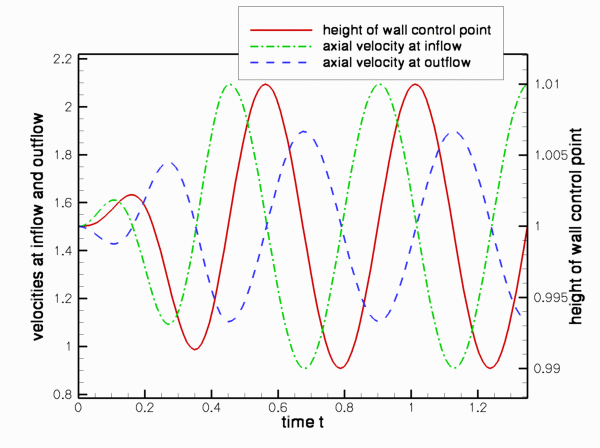Time-trace of the axial velocities at two control points in the upstream and downstream cross-sections, and the vertical position of a control point on the wall. (Re = Re St = 50, T=0.45, A=0.01.)

Finally, we comment that the plot of the perturbation velocities confirms the two-layer structure of the sloshing flows predicted in the asymptotic analysis. The sloshing flow comprises a blunt core flow region in which the flow is dominated by inertial effects while thin Stokes layers develop near the wall. Within these layers, the fluid viscosity reduces the axial velocity to zero. The carpet plot of the pressure shows that the pressure distribution is dominated by the variations induced by the oscillatory sloshing flows. For a detailed discussion of the flow structure we refer to Jensen & Heil (2003).

# Overview of the driver code

Overall, the driver code is very similar to codes developed for other moving-boundary Navier-Stokes problems, such as the driver code used to simulate the flow inside an oscillating ellipse. The present code is slightly lengthier because of the traction boundary conditions which we impose by attaching traction elements to the upstream end of the mesh. (Refer to the traction-driven Rayleigh problem for a more detailed discussion of this technique.) Also, as discussed in another example, the traction elements must be removed/re-attached before/after every mesh adaptation.

The domain is discretised by the
CollapsibleChannelMesh which employs the CollapsibleChannelDomain to provide a MacroElement - based representation of the deforming domain in terms of the GeomObject that describes the motion of the "collapsible" section of the wall (boundary 3). The sketch below illustrates the topology and the mesh deformation: As the wall deforms, the
boundaries of the MacroElements in the "collapsible" part of the Domain follow the wall motion.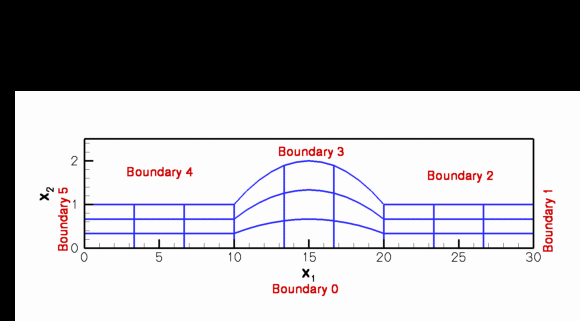Sketch of the CollapsibleChannelDomain/Mesh.

The no-slip boundary conditions on the moving wall are applied as in the oscillating ellipse problem, by executing the function FSI_functions::apply_no_slip_on_moving_wall(...) in Problem::actions_before_implicit_timestep() for all nodes that are located on boundary 3.

The following sections provide a complete annotated listing of the driver code. Most functions should already be familiar from previous examples and you may want to skip straight to the Comments and Exercises.

# The moving wall

As usual, we represent the moving wall as a GeomObject and define its shape by implementing the pure virtual function GeomObject::position(...). The arguments to the constructor specify the Eulerian coordinates of wall's left end, its undeformed length, the amplitude of the oscillations,, and the period of the oscillations. We also pass the pointer to a Time object to the constructor and store it in a private data member, to allow the position(...) functions to access the current value of the continuous time. The amplitude of the wall motion, and the period of its oscillations are stored as private data members, accessible via suitable member functions.

//===============start_of_oscillating_wall=================================
/// Straight, horizontal channel wall at \f$y=H \f$ deforms into an
/// oscillating parabola. The amplitude of the oscillation
/// \f$A \f$ and its period is \f$T \f$.
/// The position vector to a point on the wall, parametrised by
/// the Lagrangian coordinate \f$\zeta \in [0,L]\f$, is therefore given by
/// \f[ {\bf R}(\zeta,t) =
/// \left(
/// \begin{array}{c}
/// L_{up} + \zeta \\ 1
/// \end{array}
/// \right)
/// + A
/// \left(
/// \begin{array}{l}
/// - B \sin\left(\frac{2\pi}{L_{collapsible}}\zeta\right) \\ \left(
/// \frac{2}{L_{collapsible}}\right)^2 \zeta \ (L-\zeta)
/// \end{array}
/// \right)
/// \ \sin\left(\frac{2\pi t}{T}\right)
/// \ {\cal R}(t)
/// \f]
/// The parameter \f$B \f$ is zero by default. If it is set to a nonzero
/// value, the material particles on the wall also perform some
/// horizontal motion. The "ramp" function
/// \f[
/// {\cal R}(t) = \left\{
/// \begin{array}{ll}
/// \frac{1}{2}(1-\cos(\pi t/T)) & \mbox{for $t<T$} \\ 1 & \mbox{for $t \ge T$}
/// \end{array}
/// \right.
/// \f]
/// provides a "smooth" startup of the oscillation.
//=========================================================================
class OscillatingWall : public GeomObject
{
public:
/// Constructor : It's a 2D object, parametrised by
/// one Lagrangian coordinate. Arguments: height at ends, x-coordinate of
/// left end, length, amplitude of deflection, period of oscillation, and
/// pointer to time object
OscillatingWall(const double& h, const double& x_left, const double& l,
const double& a, const double& period, Time* time_pt) :
GeomObject(1,2), H(h), Length(l), X_left(x_left), A(a), B(0.0), T(period),
Time_pt(time_pt)
{}
/// Destructor: Empty
~OscillatingWall(){}
/// Access function to the amplitude
double& amplitude(){return A;}
/// Access function to the period
double& period(){return T;}

Since the GeomObject represents a moving (i.e. time-dependent) boundary, we implement both versions of the GeomObject::position(...) function: The "unsteady" version computes the position vector at the t -th previous timestep.

/// Position vector at Lagrangian coordinate zeta
/// at time level t.
void position(const unsigned& t, const Vector<double>&zeta,
Vector<double>& r) const
{
using namespace MathematicalConstants;
// Smoothly ramp up the oscillation during the first period
double ramp=1.0;
if (Time_pt->time(t)<T)
{
ramp=0.5*(1.0-cos(Pi*Time_pt->time(t)/T));
}
// Position vector
r = zeta+X_left
-B*A*sin(2.0*3.14159*zeta/Length)*
sin(2.0*Pi*(Time_pt->time(t))/T)*ramp;
r = H+A*((Length-zeta)*zeta)/pow(0.5*Length,2)*
sin(2.0*Pi*(Time_pt->time(t))/T)*ramp;
} // end of "unsteady" version

The version without additional argument computes the position vector at the present time:

/// "Current" position vector at Lagrangian coordinate zeta
void position(const Vector<double>&zeta, Vector<double>& r) const
{
position (0, zeta, r);
}

Finally, here are the various private data members:

/// Number of geometric Data in GeomObject: None.
unsigned ngeom_data() const {return 0;}
private:
/// Height at ends
double H;
/// Length
double Length;
/// x-coordinate of left end
double X_left;
/// Amplitude of oscillation
double A;
/// Relative amplitude of horizontal wall motion
double B;
/// Period of the oscillations
double T;
/// Pointer to the global time object
Time* Time_pt;
}; // end of oscillating wall

[Note: We note that the OscillatingWall class allows the wall shape to be slightly more complicated than required by (5). If the parameter B is set to a non-zero value, the material points on the wall also undergo some horizontal displacement. We will use this capability in one of the exercises in section Comments and Exercises.]

# Namespace for the "global" physical variables

As usual, we define the problem parameters in a namespace and assign default values that can be overwritten if required.

//====start_of_Global_Physical_Variables================
/// Namespace for phyical parameters
//======================================================
namespace Global_Physical_Variables
{
/// Reynolds number
double Re=50.0;
/// Womersley = Reynolds times Strouhal
double ReSt=50.0;
/// Default pressure on the left boundary
double P_up=0.0;

We also implement the function that defines the prescribed (axial) traction at the inflow boundary.

/// Traction required at the left boundary
void prescribed_traction(const double& t,
const Vector<double>& x,
const Vector<double>& n,
Vector<double>& traction)
{
traction.resize(2);
traction=P_up;
traction=0.0;
}
} // end of Global_Physical_Variables

# The driver code

As with most previous time-dependent codes, we use command line arguments to indicate if the code is run during oomph-lib's self-test. If any command line arguments are specified, we use a coarser discretisation and perform fewer timesteps.

After storing the command line arguments, we choose the number of elements in the mesh, set the lengths of the domain and choose the amplitude and period of the oscillation. The parameter values are chosen such that the wall motion resembles that in the FSI simulations shown in Fig. 5 of Jensen & Heil (2003).

//=======start_of_driver_code==================================================
/// with prescribed wall motion. Presence of command line arguments
/// indicates validation run with coarse resolution and small number of
/// timesteps.
//=============================================================================
int main(int argc, char* argv[])
{
// Store command line arguments
CommandLineArgs::setup(argc,argv);
// Reduction in resolution for validation run?
unsigned coarsening_factor=1;
if (CommandLineArgs::Argc>1)
{
coarsening_factor=4;
}
// Number of elements in the domain
unsigned nup=20/coarsening_factor;
unsigned ncollapsible=40/coarsening_factor;
unsigned ndown=40/coarsening_factor;
unsigned ny=16/coarsening_factor;
// Length of the domain
double lup=5.0;
double lcollapsible=10.0;
double ldown=10.0;
double ly=1.0;
// Initial amplitude of the wall deformation
// Period of oscillation
double period=0.45;

We set the (non-dimensional) upstream pressure to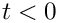, so that in the absence of any wall oscillation, the steady flow through the channel is Poiseuille flow,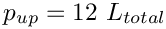; see Comments and Exercises.

// Pressure/applied traction on the left boundary: This is consistent with
Global_Physical_Variables::P_up=12.0*(lup+lcollapsible+ldown);

Next, we specify the output directory and build the problem with refineable 2D Crouzeix Raviart Elements.

//Set output directory
DocInfo doc_info;
doc_info.set_directory("RESLT");
// Open a trace file
ofstream trace_file;
char filename;
sprintf(filename,"%s/trace.dat",doc_info.directory().c_str());
trace_file.open(filename);
// Build the problem with Crouzeix Raviart Elements
CollapsibleChannelProblem<RefineableQCrouzeixRaviartElement<2> >
problem(nup, ncollapsible, ndown, ny,
lup, lcollapsible, ldown, ly,
amplitude,period);

Next we set up the time-stepping parameters for a simulation of three periods of the oscillation, performed with 40 timesteps per period. Fewer timesteps are performed if the code is run in self-test mode.

// Number of timesteps per period
unsigned nsteps_per_period=40;
// Number of periods
unsigned nperiod=3;
// Number of timesteps (reduced for validation)
unsigned nstep=nsteps_per_period*nperiod;
if (CommandLineArgs::Argc>1)
{
nstep=3;
}
//Timestep:
double dt=period/double(nsteps_per_period);
// Start time
double t_min=0.0;

We initialise the timestepper and set the initial conditions before documenting the initial condition.

// Initialise timestep and set initial conditions
problem.time_pt()->time()=t_min;
problem.initialise_dt(dt);
problem.set_initial_condition();
// Output the initial solution
problem.doc_solution(doc_info, trace_file);
// Step number
doc_info.number()++;

Next we set the error targets for the adaptive mesh refinement; a smaller target error is used when the code is run in self-test mode to ensure that some mesh refinement is performed during the first few timesteps.

// Set targets for spatial adaptivity
problem.bulk_mesh_pt()->max_permitted_error()=1.0e-3;
problem.bulk_mesh_pt()->min_permitted_error()=1.0e-5;
// Overwrite with reduced targets for validation run to force
// some refinement during the first few timesteps
if (CommandLineArgs::Argc>1)
{
problem.bulk_mesh_pt()->max_permitted_error()=1.0e-4;
problem.bulk_mesh_pt()->min_permitted_error()=1.0e-6;
}

The timestepping loop itself is identical to that used in other time-dependent driver codes with adaptive mesh refinement. During the first timestep, an arbitrary number of spatial adaptations may be performed, as the initial condition can be re-assigned on the refined mesh. (This is indicated by setting the boolean flag first to true when calling the spatially adaptive, unsteady Newton solver.) During subsequent timesteps the need to interpolate the history values onto the refined mesh limits the benefits of repeated mesh adaptations and we limit the number of spatial adaptations per timestep to 1.

// First timestep: We may re-assign the initial condition
bool first=true;
// Max. number of adaptations during first timestep
// Timestepping loop
for (unsigned istep=0;istep<nstep;istep++)
{
// Solve the problem
// Outpt the solution
problem.doc_solution(doc_info, trace_file);
// Step number
doc_info.number()++;
// We've done one step: Don't re-assign the initial conditions
// and limit the number of adaptive mesh refinements to one
// per timestep.
first=false;
}
trace_file.close();
} //end of driver code

# The problem class

As usual, we template the problem class by the element type and provide an access functions to the "bulk" Navier-Stokes mesh.

//=======start_of_problem_class=======================================
/// Problem class
//====================================================================
template <class ELEMENT>
class CollapsibleChannelProblem : public Problem
{
public :
/// Constructor : the arguments are the number of elements,
/// the length of the domain and the amplitude and period of
/// the oscillations
CollapsibleChannelProblem(const unsigned& nup,
const unsigned& ncollapsible,
const unsigned& ndown,
const unsigned& ny,
const double& lup,
const double& lcollapsible,
const double& ldown,
const double& ly,
const double& amplitude,
const double& period);
/// Empty destructor
~CollapsibleChannelProblem() {}
/// Access function for the specific mesh
RefineableCollapsibleChannelMesh<ELEMENT>* bulk_mesh_pt()
{
// Upcast from pointer to the Mesh base class to the specific
// element type that we're using here.
return dynamic_cast<RefineableCollapsibleChannelMesh<ELEMENT>*>
(Bulk_mesh_pt);

No action is needed before or after solving, so the pure virtual functions Problem::actions_before_newton_solve() and Problem::actions_after_newton_solve() can remain empty.

/// Update the problem specs before solve (empty)
void actions_before_newton_solve(){}
/// Update the problem after solve (empty)
void actions_after_newton_solve(){}

We will use the function Problem::actions_before_implicit_timestep() to update the no-slip boundary conditions on the moving wall before each timestep and employ Problem::actions_before_adapt() and Problem::actions_after_adapt() to wipe and rebuild the mesh of prescribed traction elements each time a mesh adaptation is performed. The functions Problem::doc_solution(...) and Problem::set_initial_condition() will do what they say...

/// Update the velocity boundary condition on the moving wall
void actions_before_implicit_timestep();
/// Actions before adapt: Wipe the mesh of prescribed traction elements
/// Actions after adapt: Rebuild the mesh of prescribed traction elements
/// Apply initial conditions
void set_initial_condition();
/// Doc the solution
void doc_solution(DocInfo& doc_info, ofstream& trace_file);

The private helper functions create_traction_elements(...) and delete_traction_elements() attach and remove the traction elements from the upstream boundary of the "bulk" Navier-Stokes mesh.

private :
/// Create the prescribed traction elements on boundary b
/// of the bulk mesh and stick them into the surface mesh.
void create_traction_elements(const unsigned &b,
Mesh* const &bulk_mesh_pt,
Mesh* const &surface_mesh_pt);
/// Delete prescribed traction elements from the surface mesh
void delete_traction_elements(Mesh* const &surface_mesh_pt);

The private member data contains the geometric parameters as well as the pointer to the GeomObject that describes the moving wall.

/// Number of elements in the x direction in the upstream part of the channel
unsigned Nup;
/// Number of elements in the x direction in the "collapsible"
/// part of the channel
unsigned Ncollapsible;
/// Number of elements in the x direction in the downstream part of the channel
unsigned Ndown;
/// Number of elements across the channel
unsigned Ny;
/// x-length in the upstream part of the channel
double Lup;
/// x-length in the "collapsible" part of the channel
double Lcollapsible;
/// x-length in the downstream part of the channel
double Ldown;
/// Transverse length
double Ly;
/// Pointer to the geometric object that parametrises the "collapsible" wall
OscillatingWall* Wall_pt;

Further private member data includes pointers to the "bulk" mesh and the surface mesh that contains the traction elements, and pointers to control nodes in the in- and outflow cross-sections.

/// Pointer to the "bulk" mesh
RefineableCollapsibleChannelMesh<ELEMENT>* Bulk_mesh_pt;
/// Pointer to the "surface" mesh that contains the applied traction
/// elements
Mesh* Surface_mesh_pt;
/// Pointer to the left control node
Node* Left_node_pt;
/// Pointer to right control node
Node* Right_node_pt;
}; // end of problem class

# The problem constructor

The arguments passed to the problem constructor specify the number of elements and lengths of the various parts of the channel, as well as the amplitude and period of the wall oscillations.

We store the parameters in the problem's private member data and increase the maximum permitted residual for the Newton iteration.

//===start_of_constructor=======================================
/// Constructor for the collapsible channel problem
//===============================================================
template <class ELEMENT>
CollapsibleChannelProblem<ELEMENT>::CollapsibleChannelProblem(
const unsigned& nup,
const unsigned& ncollapsible,
const unsigned& ndown,
const unsigned& ny,
const double& lup,
const double& lcollapsible,
const double& ldown,
const double& ly,
const double& amplitude,
const double& period)
{
// Number of elements
Nup=nup;
Ncollapsible=ncollapsible;
Ndown=ndown;
Ny=ny;
// Lengths of domain
Lup=lup;
Lcollapsible=lcollapsible;
Ldown=ldown;
Ly=ly;
// Overwrite maximum allowed residual to accomodate possibly
// poor initial guess for solution
Problem::Max_residuals=10000;

We continue by building the BDF<2> timestepper and pass a pointer to it to the Problem

// Allocate the timestepper -- this constructs the Problem's
// time object with a sufficient amount of storage to store the
// previous timsteps.

Next, we create the GeomObject that represents the oscillating wall, and pass a pointer to it to the constructor of the CollapsibleChannelMesh

//Create the geometric object that represents the wall
Wall_pt=new OscillatingWall(height, x_left, length, amplitude, period,
time_pt());
//Build mesh
Bulk_mesh_pt = new RefineableCollapsibleChannelMesh<ELEMENT>(
nup, ncollapsible, ndown, ny,
lup, lcollapsible, ldown, ly,
Wall_pt,
time_stepper_pt());

We create a second mesh to store the applied traction elements and attach them to the inflow boundary (boundary 5) of the "bulk" fluid mesh, using the function create_traction_elements(...). Both submeshes are then combined into a global mesh.

// Create "surface mesh" that will contain only the prescribed-traction
// elements at the inflow. The default constructor just creates the mesh
// without giving it any elements, nodes, etc.
Surface_mesh_pt = new Mesh;
// Create prescribed-traction elements from all elements that are
// adjacent to boundary 5 (inflow boundary), and add them to the surface mesh.
create_traction_elements(5,Bulk_mesh_pt,Surface_mesh_pt);
// Add the two sub meshes to the problem
// Combine all submeshes added so far into a single Mesh
build_global_mesh();

We create the spatial error estimator for the fluid mesh and loop over the various elements to set the pointers to the relevant physical parameters, first for the Navier-Stokes elements in the bulk mesh,

//Set errror estimator for bulk mesh
Z2ErrorEstimator* error_estimator_pt=new Z2ErrorEstimator;
dynamic_cast<RefineableCollapsibleChannelMesh<ELEMENT>*>
(Bulk_mesh_pt)->spatial_error_estimator_pt()=error_estimator_pt;
// Loop over the elements to set up element-specific
// things that cannot be handled by constructor
unsigned n_element=Bulk_mesh_pt->nelement();
for(unsigned e=0;e<n_element;e++)
{
// Upcast from GeneralisedElement to the present element
ELEMENT* el_pt = dynamic_cast<ELEMENT*>(Bulk_mesh_pt->element_pt(e));
//Set the Reynolds number
el_pt->re_pt() = &Global_Physical_Variables::Re;
// Set the Womersley number
el_pt->re_st_pt() = &Global_Physical_Variables::ReSt;
} // end loop over bulk elements

and then for the applied traction elements in the surface mesh:

// Loop over the traction elements to pass pointer to prescribed
// traction function
unsigned n_el=Surface_mesh_pt->nelement();
for(unsigned e=0;e<n_el;e++)
{
// Upcast from GeneralisedElement to NavierStokes traction element
NavierStokesTractionElement<ELEMENT> *el_pt =
dynamic_cast< NavierStokesTractionElement<ELEMENT>*>(
Surface_mesh_pt->element_pt(e));
// Set the pointer to the prescribed traction function
el_pt->traction_fct_pt() = &Global_Physical_Variables::prescribed_traction;
} // end loop over applied traction elements

We apply the boundary conditions and pin the velocity on the relevant mesh boundaries:

• both axial and transverse velocities are pinned along the bottom and the top boundaries (boundaries 0, 2, 3 and 4).
• the transverse velocities are pinned along the in- and outflow boundaries (boundaries 1 and 5).
//Pin the velocity on the boundaries
//x and y-velocities pinned along boundary 0 (bottom boundary) :
unsigned ibound=0;
unsigned num_nod= bulk_mesh_pt()->nboundary_node(ibound);
for (unsigned inod=0;inod<num_nod;inod++)
{
for(unsigned i=0;i<2;i++)
{
bulk_mesh_pt()->boundary_node_pt(ibound, inod)->pin(i);
}
}
//x and y-velocities pinned along boundary 2, 3, 4 (top boundaries) :
for(unsigned ib=2;ib<5;ib++)
{
num_nod= bulk_mesh_pt()->nboundary_node(ib);
for (unsigned inod=0;inod<num_nod;inod++)
{
for(unsigned i=0;i<2;i++)
{
bulk_mesh_pt()->boundary_node_pt(ib, inod)->pin(i);
}
}
}
//y-velocity pinned along boundary 1 (right boundary):
ibound=1;
num_nod= bulk_mesh_pt()->nboundary_node(ibound);
for (unsigned inod=0;inod<num_nod;inod++)
{
bulk_mesh_pt()->boundary_node_pt(ibound, inod)->pin(1);
}
//y-velocity pinned along boundary 5 (left boundary):
ibound=5;
num_nod= bulk_mesh_pt()->nboundary_node(ibound);
for (unsigned inod=0;inod<num_nod;inod++)
{
bulk_mesh_pt()->boundary_node_pt(ibound, inod)->pin(1);
}// end of pin_velocity

We select two control nodes on the inflow and outflow boundaries to document the velocities.

//Select control nodes "half way" up the inflow/outflow cross-sections
//--------------------------------------------------------------------
// Left boundary
ibound=5;
num_nod= bulk_mesh_pt()->nboundary_node(ibound);
unsigned control_nod=num_nod/2;
Left_node_pt= bulk_mesh_pt()->boundary_node_pt(ibound, control_nod);
// Right boundary
ibound=1;
num_nod= bulk_mesh_pt()->nboundary_node(ibound);
control_nod=num_nod/2;
Right_node_pt= bulk_mesh_pt()->boundary_node_pt(ibound, control_nod);

Finally, we set up the equation numbering scheme.

// Setup equation numbering scheme
cout <<"Number of equations: " << assign_eqn_numbers() << std::endl;
} //end of constructor

# Post processing

The function doc_solution(...) documents the results, and records the time-trace of the axial velocities at the two control nodes and the position of the midpoint on the oscillating wall.

//====start_of_doc_solution===================================================
/// Doc the solution
//============================================================================
template <class ELEMENT>
void CollapsibleChannelProblem<ELEMENT>:: doc_solution(DocInfo& doc_info,
ofstream& trace_file)
{
ofstream some_file;
char filename;
// Number of plot points
unsigned npts;
npts=5;
// Output solution
sprintf(filename,"%s/soln%i.dat",doc_info.directory().c_str(),
doc_info.number());
some_file.open(filename);
bulk_mesh_pt()->output(some_file,npts);
some_file.close();
// Get the position of the midpoint on the geometric object
Vector<double> zeta(1);
zeta=0.5*Lcollapsible;
Vector<double> wall_point(2);
Wall_pt->position(zeta,wall_point);
// Write trace file
trace_file << time_pt()->time() << " "
<< wall_point << " "
<< Left_node_pt->value(0) << " "
<< Right_node_pt->value(0) << " "
<< std::endl;
// Output wall shape
sprintf(filename,"%s/wall%i.dat",doc_info.directory().c_str(),
doc_info.number());
some_file.open(filename);
unsigned nplot=100;
for (unsigned i=0;i<nplot;i++)
{
zeta=double(i)/double(nplot-1)*Lcollapsible;
Wall_pt->position(zeta,wall_point);
some_file << wall_point << " "
<< wall_point << std::endl;
}
some_file.close();
} // end_of_doc_solution

# Creation of the traction elements

The creation of the applied traction elements follows the usual pattern, explained in detail elsewhere: We loop over the elements in the fluid mesh that are adjacent to the specified mesh boundary, and build the corresponding traction elements, which are added to the surface mesh.

//==========start_of_create_traction_elements==================================
/// Create the traction elements
//============================================================================
template <class ELEMENT>
void CollapsibleChannelProblem<ELEMENT>::create_traction_elements(
const unsigned &b, Mesh* const &bulk_mesh_pt, Mesh* const &surface_mesh_pt)
{
// How many bulk elements are adjacent to boundary b?
unsigned n_element = bulk_mesh_pt->nboundary_element(b);
// Loop over the bulk elements adjacent to boundary b
for(unsigned e=0;e<n_element;e++)
{
// Get pointer to the bulk element that is adjacent to boundary b
ELEMENT* bulk_elem_pt = dynamic_cast<ELEMENT*>
(bulk_mesh_pt->boundary_element_pt(b,e));
//What is the index of the face of element e that lies along boundary b
int face_index = bulk_mesh_pt->face_index_at_boundary(b,e);
// Build the corresponding prescribed-traction element
NavierStokesTractionElement<ELEMENT>* traction_element_pt =
new NavierStokesTractionElement<ELEMENT>(bulk_elem_pt,face_index);
//Add the prescribed-flux element to the surface mesh
} //end of loop over bulk elements adjacent to boundary b
} // end of create_traction_elements

# Delete the traction elements

Since the "bulk" elements that the applied traction elements are attached to may disappear during mesh adaptation, we delete all traction elements before the adaptation and re-attach them afterwards. The deletion is performed by the following member function. Note that the surface mesh that contains the traction elements is not deleted, as this would also delete the associated nodes which are shared with the corresponding bulk elements.

//============start_of_delete_traction_elements==============================
/// Delete traction elements and wipe the surface mesh
//=======================================================================
template<class ELEMENT>
void CollapsibleChannelProblem<ELEMENT>::
delete_traction_elements(Mesh* const &surface_mesh_pt)
{
// How many surface elements are in the surface mesh
unsigned n_element = surface_mesh_pt->nelement();
// Loop over the surface elements
for(unsigned e=0;e<n_element;e++)
{
// Kill surface element
delete surface_mesh_pt->element_pt(e);
}
// Wipe the mesh
surface_mesh_pt->flush_element_and_node_storage();
} // end of delete_traction_elements

# Apply the initial conditions

Initial conditions are applied as usual. We start by confirming that the timestepper is a member of the BDF family and therefore operates on history values that represent the solution at previous timesteps. We assign the previous nodal positions and velocities at all nodes, assuming that for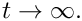the wall is at rest and the flow field is given by steady Poiseuille flow.

//=======start_of_apply_initial_conditions===================================
/// Apply initial conditions: Impulsive start from steady Poiseuille flow
//============================================================================
template <class ELEMENT>
void CollapsibleChannelProblem<ELEMENT>::set_initial_condition()
{
// Check that timestepper is from the BDF family
if (time_stepper_pt()->type()!="BDF")
{
std::ostringstream error_stream;
error_stream
<< "Timestepper has to be from the BDF family!\n"
<< "You have specified a timestepper from the "
<< time_stepper_pt()->type() << " family" << std::endl;
throw OomphLibError(error_stream.str(),
OOMPH_CURRENT_FUNCTION,
OOMPH_EXCEPTION_LOCATION);
}
// Update the mesh
bulk_mesh_pt()->node_update();
// Loop over the nodes to set initial guess everywhere
unsigned num_nod = bulk_mesh_pt()->nnode();
for (unsigned n=0;n<num_nod;n++)
{
// Get nodal coordinates
Vector<double> x(2);
x=bulk_mesh_pt()->node_pt(n)->x(0);
x=bulk_mesh_pt()->node_pt(n)->x(1);
bulk_mesh_pt()->node_pt(n)->set_value(0,6.0*(x/Ly)*(1.0-(x/Ly)));
bulk_mesh_pt()->node_pt(n)->set_value(1,0.0);
}
bulk_mesh_pt()->assign_initial_values_impulsive();
} // end of set_initial_condition

# Actions before the timestep

Before each timestep, we update the nodal positions in the fluid mesh, and apply the no-slip condition to each node on mesh boundary 3.

//=====start_of_actions_before_implicit_timestep==============================
/// Execute the actions before timestep: Update the velocity
/// boundary condition on the moving wall
//============================================================================
template <class ELEMENT>
void CollapsibleChannelProblem<ELEMENT>::actions_before_implicit_timestep()
{
// Update the domain shape
bulk_mesh_pt()->node_update();
// Moving wall: No slip; this implies that the velocity needs
// to be updated in response to wall motion
unsigned ibound=3;
unsigned num_nod=bulk_mesh_pt()->nboundary_node(ibound);
for (unsigned inod=0;inod<num_nod;inod++)
{
// Which node are we dealing with?
Node* node_pt=bulk_mesh_pt()->boundary_node_pt(ibound,inod);
// Apply no slip
FSI_functions::apply_no_slip_on_moving_wall(node_pt);
}
} //end of actions_before_implicit_timestep

# Actions before the mesh adaptation

As discussed above, we delete the applied traction elements before performing any mesh adaptation and then rebuild the global mesh.

/// Actions before adapt: Wipe the mesh of prescribed traction elements
//========================================================================
template<class ELEMENT>
{
// Kill the traction elements and wipe surface mesh
delete_traction_elements(Surface_mesh_pt);
// Rebuild the global mesh.
rebuild_global_mesh();

# Actions before the mesh adaptation

Once the mesh has been adapted, we (re-)create the prescribed traction elements and rebuild the global mesh. We also have to pass the pointers to prescribed traction function to the newly created traction elements.

/// Actions after adapt: Rebuild the mesh of prescribed traction elements
//========================================================================
template<class ELEMENT>
{
// Create prescribed-flux elements from all elements that are
create_traction_elements(5,Bulk_mesh_pt,Surface_mesh_pt);
// Rebuild the global mesh
rebuild_global_mesh();
// Loop over the traction elements to pass pointer to prescribed traction function
unsigned n_element=Surface_mesh_pt->nelement();
for(unsigned e=0;e<n_element;e++)
{
// Upcast from GeneralisedElement to NavierStokesTractionElement element
NavierStokesTractionElement<ELEMENT> *el_pt =
dynamic_cast<NavierStokesTractionElement<ELEMENT>*>(
Surface_mesh_pt->element_pt(e));
// Set the pointer to the prescribed traction function
el_pt->traction_fct_pt() = &Global_Physical_Variables::prescribed_traction;
}

1. Check the non-dimensionalisation of the governing equations and confirm that a (non-dimensional) upstream pressure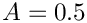is required to drive the steady Poiseuille flow specified by (3) through the static, undeformed channel. Use this to "validate" (well, "plausibility-check", anyway...) the code by setting the amplitude of the wall oscillation to zero.
2. Double the upstream pressure while keeping the amplitude of the wall oscillation at zero and confirm that the flow accelerates until it (asymptotically) approaches Poiseuille flow with twice the initial flowrate as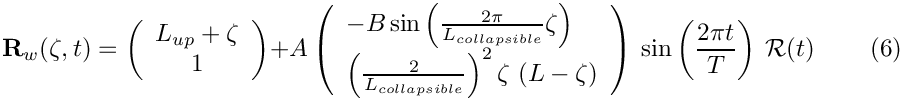3. The flow field has the largest velocity gradients in the thin Stokes layers near the wall, causing the automatic mesh adaptation procedure to refine the mesh pre-dominantly in these regions. To facilitate the resolution of such layers the CollapsibleChannelDomain and CollapsibleChannelMesh allow the specification of a mapping [0,1] -> [0,1] that redistributes the nodal points in the vertical direction so that the elements near the wall become more squashed. By default the "boundary-layer squash function" is the identity but it may be overloaded by specifying a function pointer to an alternative function. The driver code already includes a demonstration of this capability which may be activated by compiling the driver code with -DUSE_BL_SQUASH_FCT. This activates the code segment
#ifdef USE_BL_SQUASH_FCT
// Set a non-trivial boundary-layer-squash function...
Bulk_mesh_pt->bl_squash_fct_pt() = &BL_Squash::squash_fct;
// ... and update the nodal positions accordingly
Bulk_mesh_pt->node_update();
#endif
double squash_fct(const double &s)
Mapping [0,1] -> [0,1] that re-distributes nodal points across the channel width.
in the Problem constructor. The "squash function" used for this example is defined in the following namespace:
//==========start_of_BL_Squash =========================================
/// Namespace to define the mapping [0,1] -> [0,1] that re-distributes
/// nodal points across the channel width.
//======================================================================
namespace BL_Squash
{
/// Boundary layer width
double Delta=0.1;
/// Fraction of points in boundary layer
double Fract_in_BL=0.5;
/// Mapping [0,1] -> [0,1] that re-distributes
/// nodal points across the channel width
double squash_fct(const double& s)
{
// Default return
double y=s;
if (s<0.5*Fract_in_BL)
{
y=Delta*2.0*s/Fract_in_BL;
}
else if (s>1.0-0.5*Fract_in_BL)
{
}
else
{
y=(1.0-2.0*Delta)/(1.0-Fract_in_BL)*s+
}
return y;
}
}// end of BL_Squash
Namespace to define the mapping [0,1] -> [0,1] that re-distributes nodal points across the channel wi...
double Delta
Boundary layer width.
double Fract_in_BL
Fraction of points in boundary layer.
With this function 50% of the nodal points in the vertical direction are located within two boundary-layer regions which occupy 2 x 10% of the channel's width. The figure below shows the element shapes for a (coarse) initial mesh that is used in the validation run, with and without the boundary-layer squashing function: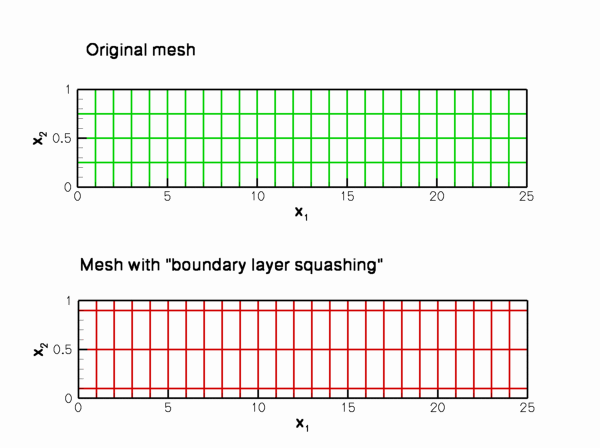Coarse initial meshes with and without the boundary-layer squash function.
Confirm that if this "squashing function" is applied to the mesh that is used during the non-self-test runs (this mesh has 16 x larger number of elements than the meshes shown above), the quality of the computed solution improves so much that no subsequent mesh adaptation is required.
4. The flow structures observed during the small-amplitude oscillations (shown in the animation at the beginning of this document) are in perfect agreement with the structures predicted by Jensen & Heil's (2003) asymptotic analysis. As an exercise, increase the amplitude of the wall oscillation (to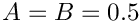, say) to confirm that the flow-structure predicted by the theory (which is strictly applicable only for small-amplitude
oscillations) also provides an excellent description of of the system's behaviour during large-amplitude oscillations with more complicated wall motions.

For instance, the figure below shows a snapshot of the the animation of the computational results for an oscillation in which the wall undergoes a more complicated motion, described by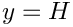for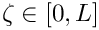. For these parameter values, the wall performs a large-amplitude oscillation in the course of which material particles are not only displaced vertically but also in the horizontal direction. Nevertheless, the flow generated by the moving wall may be described as arising from the superposition of Poiseuille flow and an axial sloshing motion, the latter obviously having a much larger amplitude than in the previous case. The
animation of the flow field shows that more complex local flow features develop briefly whenever the flow separates from the wall. However, the appearance of these features does not change the macroscopic behaviour of the flow.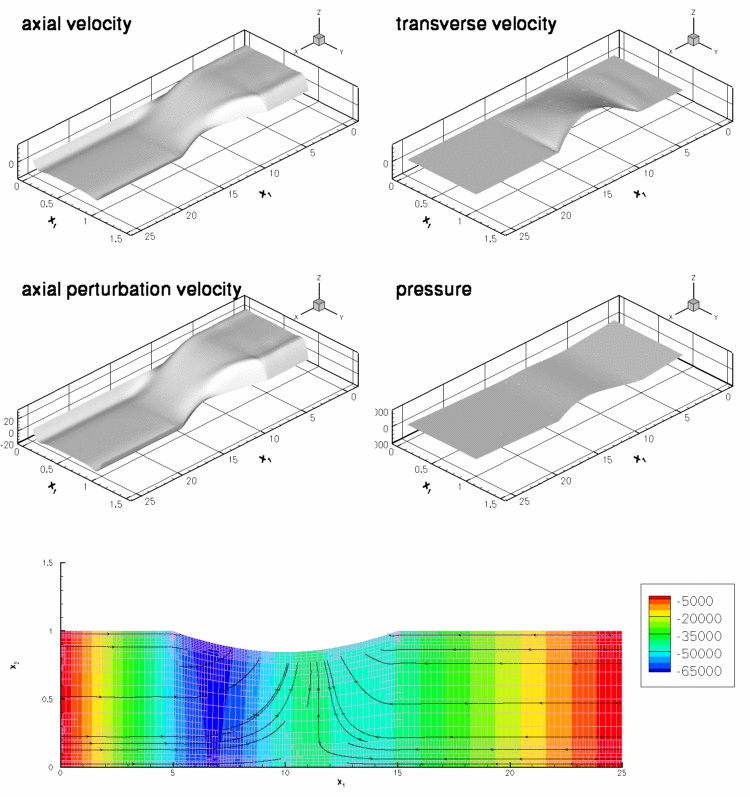Flow field for a large-amplitude wall motion. Re=ReSt=50; A=B=0.5; T=0.45.